# RD Sharma Solutions Class 12 Straight Line In Space Exercise 28.5

RD Sharma Solutions for Class 12 Maths Chapter 28 Straight Line in Space Exercise 28.5, is provided here for students prepare well for their board exams. Tutors have designed the solutions in a unique and simple method where any student can grasp the topics clearly. The RD Sharma Solutions to this chapter are readily available in the pdf format, which can be downloaded by the students easily from the links provided below and can start practising offline to score good marks in their board exams.

## Download PDF of Rd Sharma Solution for Class 12 Maths Chapter 28 Exercise 5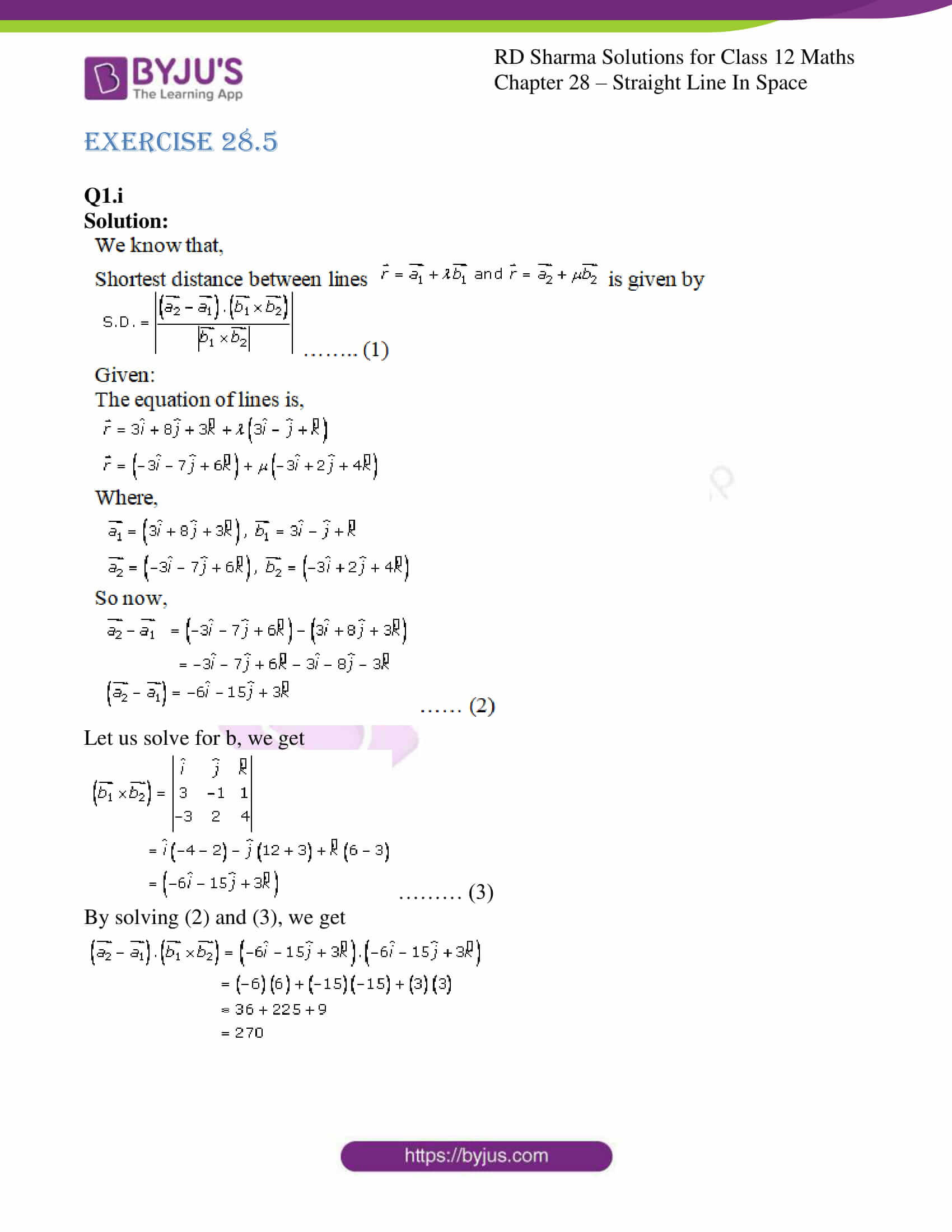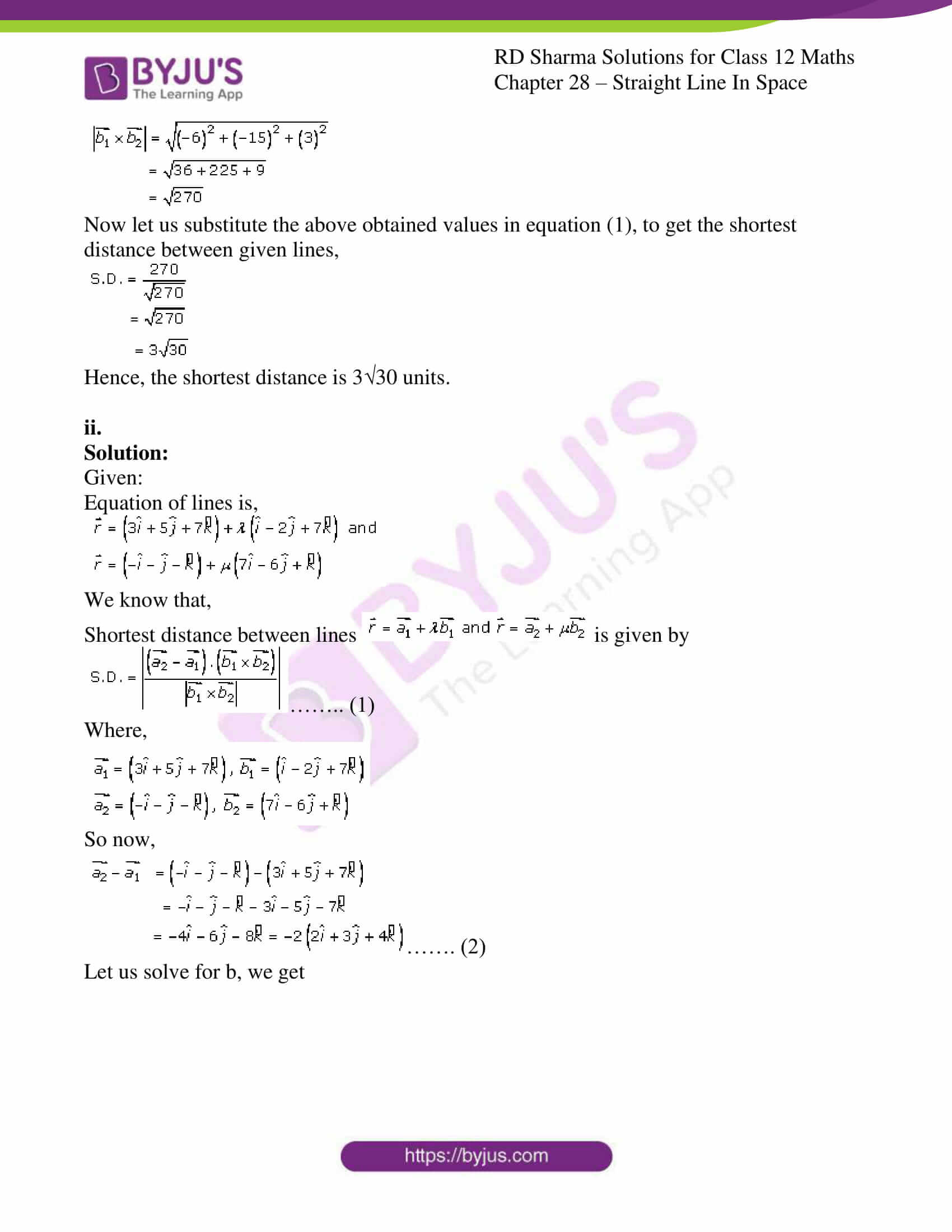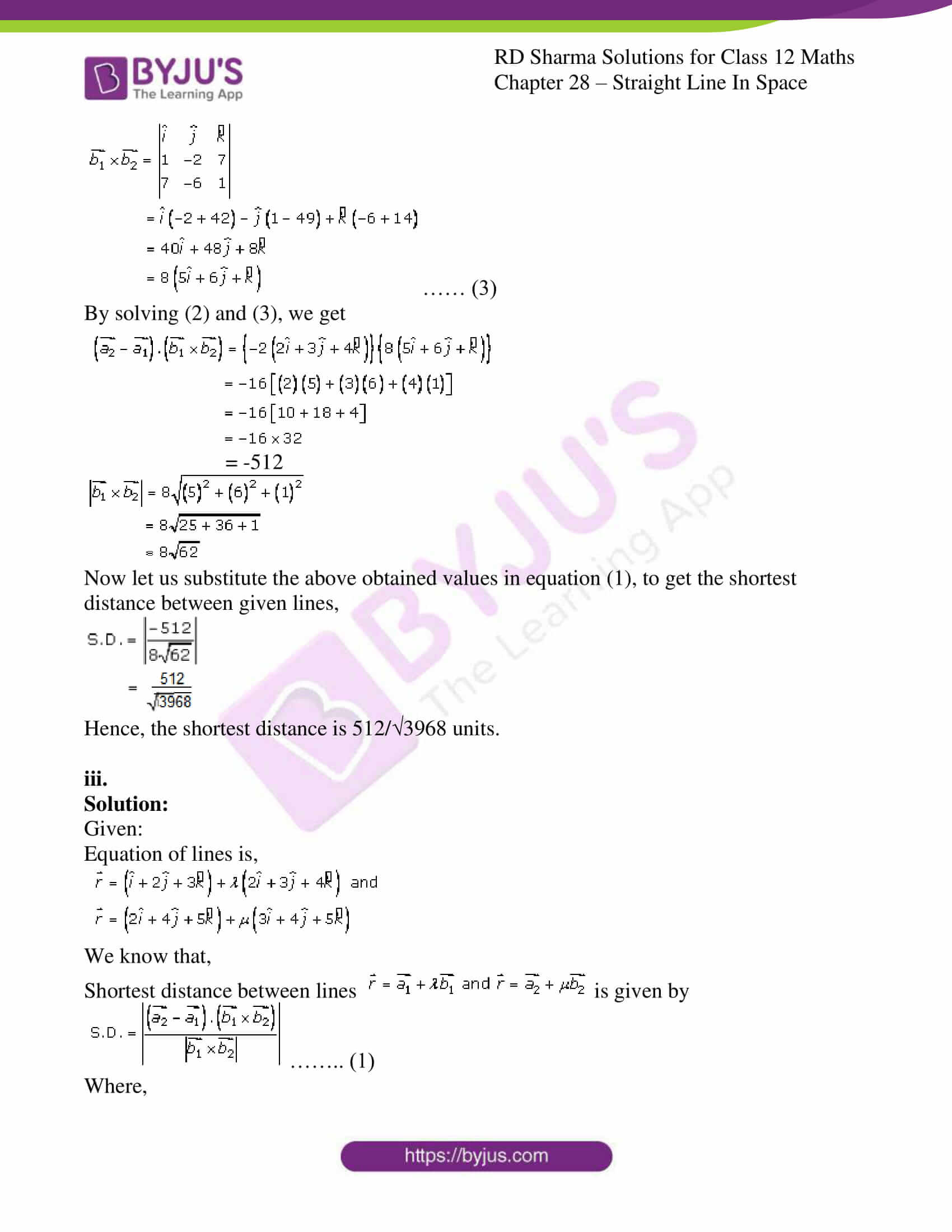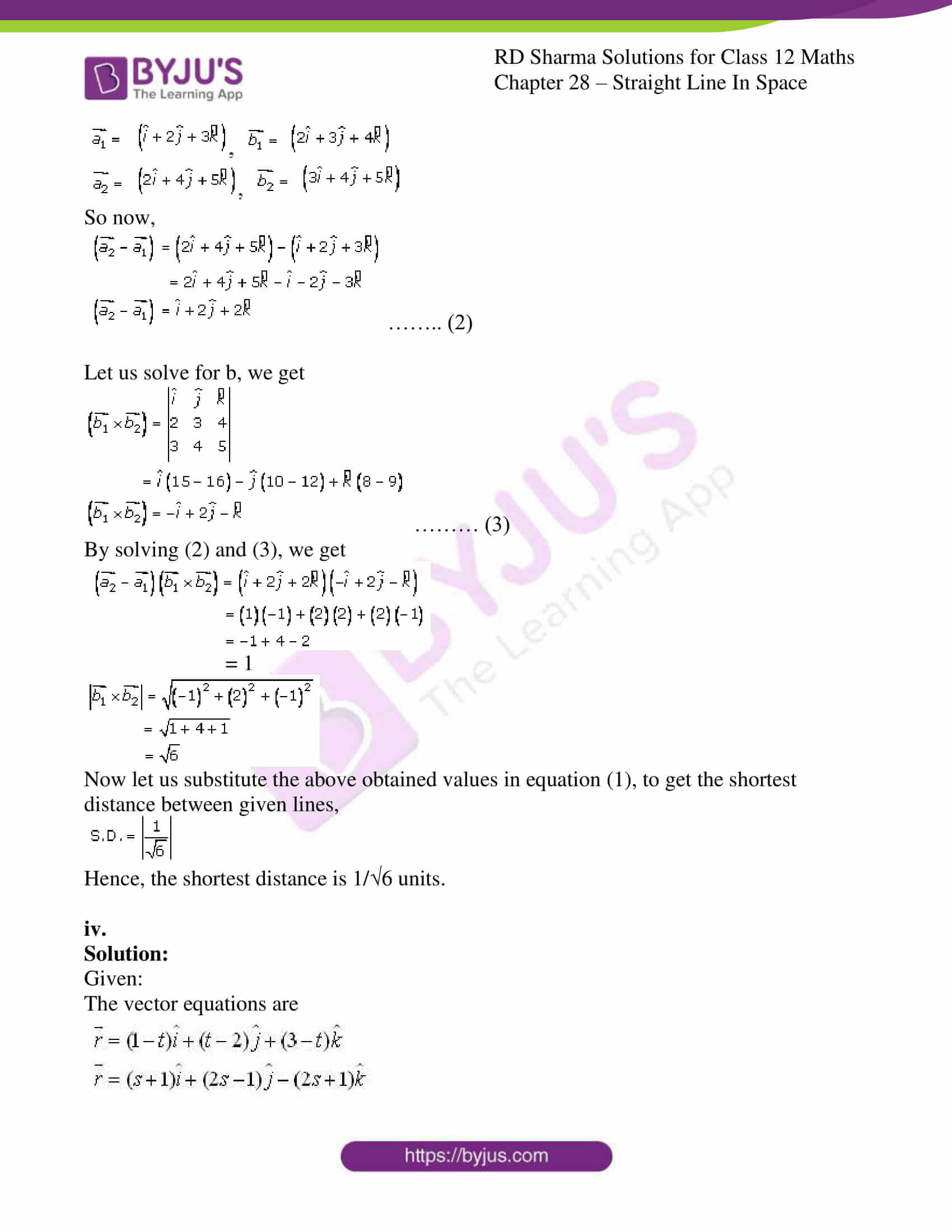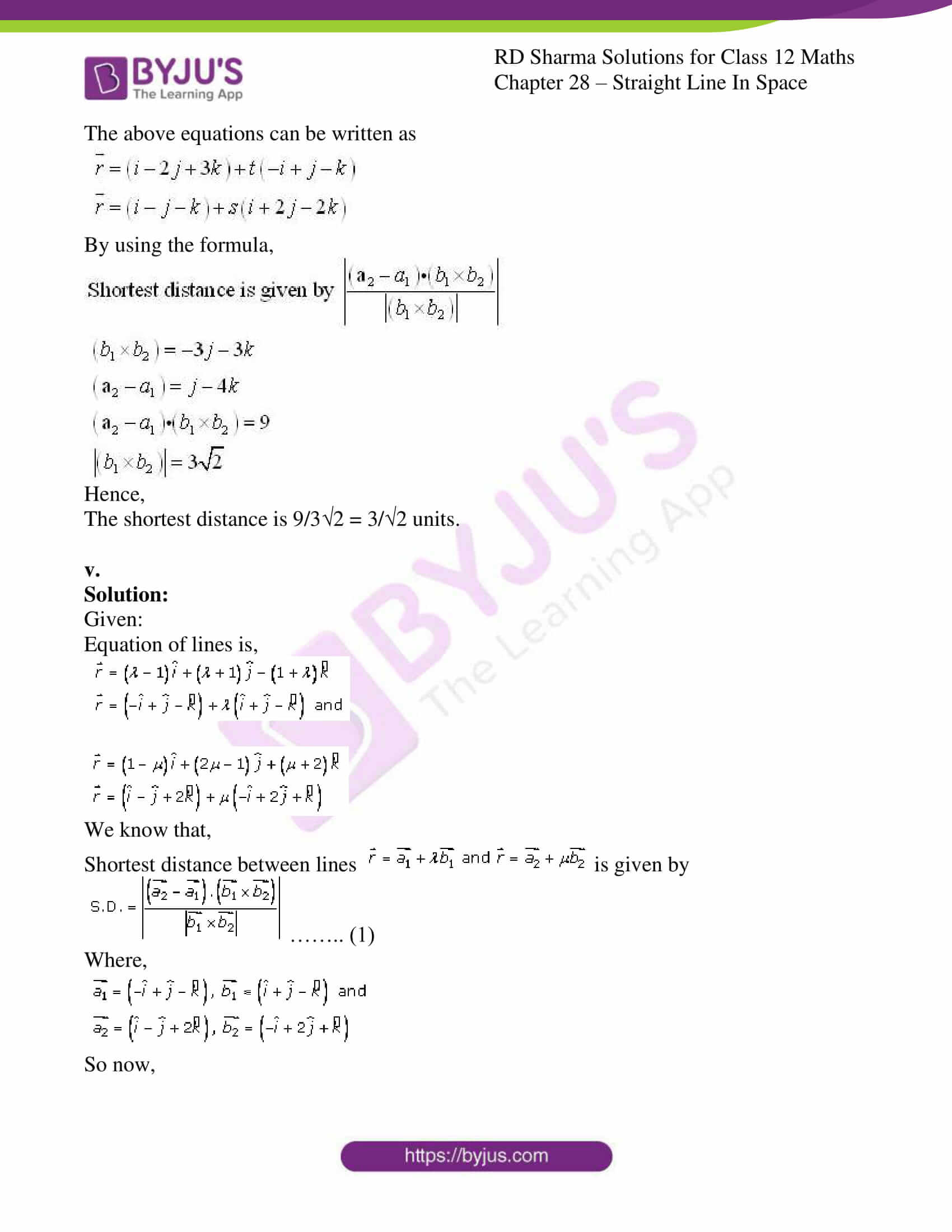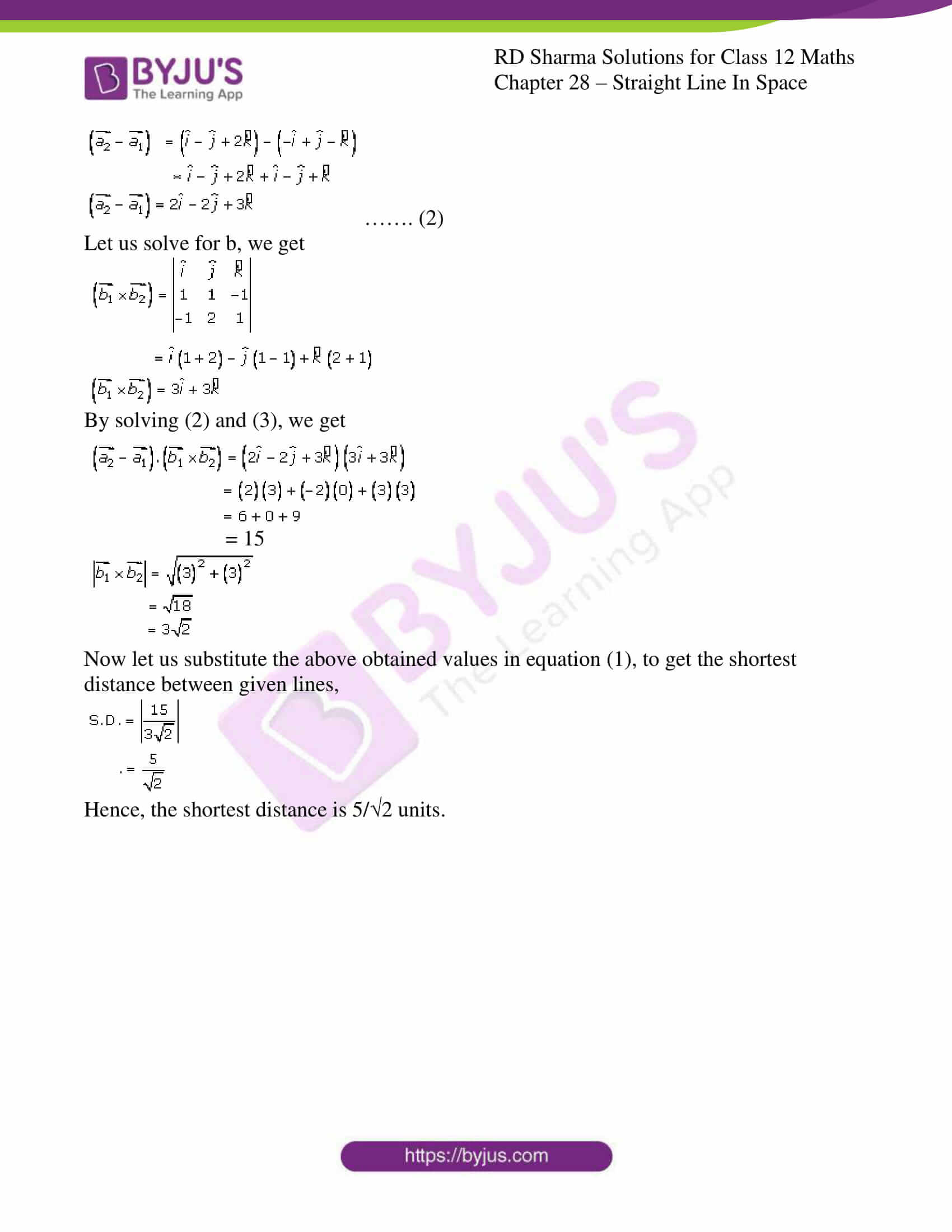### Access Answers for Rd Sharma Solution Class 12 Maths Chapter 28 Exercise 5

Q1.i

Solution: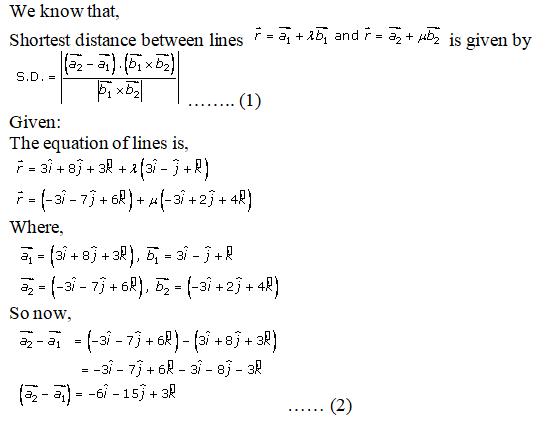Let us solve for b, we get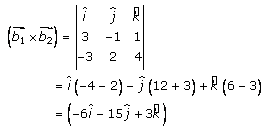……… (3)

By solving (2) and (3), we get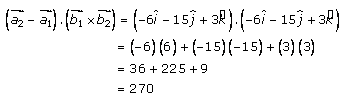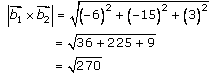Now let us substitute the above obtained values in equation (1), to get the shortest distance between given lines,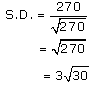Hence, the shortest distance is 3√30 units.

ii.

Solution:

Given:

Equation of lines is,We know that,

Shortest distance between lines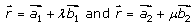is given by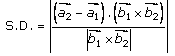…….. (1)

Where,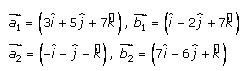So now,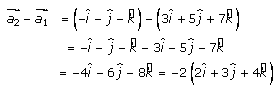……. (2)

Let us solve for b, we get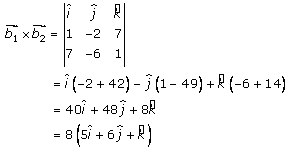…… (3)

By solving (2) and (3), we get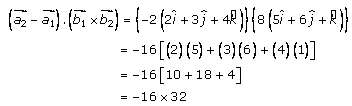= -512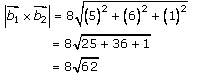Now let us substitute the above obtained values in equation (1), to get the shortest distance between given lines,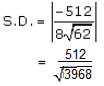Hence, the shortest distance is 512/√3968 units.

iii.

Solution:

Given:

Equation of lines is,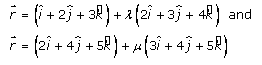We know that,

Shortest distance between linesis given by…….. (1)

Where,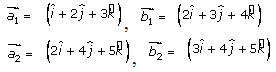So now,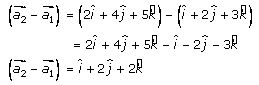…….. (2)

Let us solve for b, we get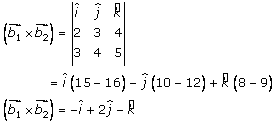……… (3)

By solving (2) and (3), we get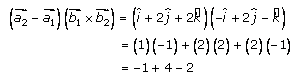= 1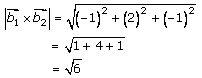Now let us substitute the above obtained values in equation (1), to get the shortest distance between given lines,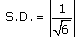Hence, the shortest distance is 1/√6 units.

iv.

Solution:

Given:

The vector equations are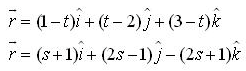The above equations can be written as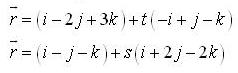By using the formula,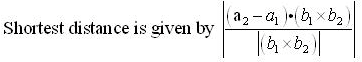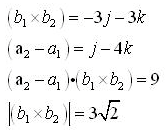Hence,

The shortest distance is 9/3√2 = 3/√2 units.

v.

Solution:

Given:

Equation of lines is,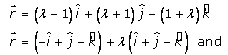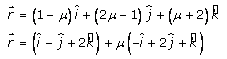We know that,

Shortest distance between linesis given by…….. (1)

Where,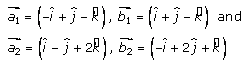So now,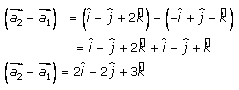……. (2)

Let us solve for b, we get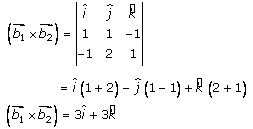By solving (2) and (3), we get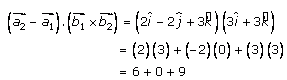= 15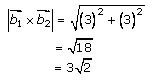Now let us substitute the above obtained values in equation (1), to get the shortest distance between given lines,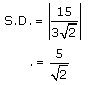Hence, the shortest distance is 5/√2 units.